# Introduction to R - 5th lesson (statistics)

Nathalie Villa-Vialaneix - http://www.nathalievilla.org
September 14-16th, 2015

Master TIDE, Université Paris 1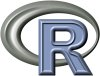## Probability distribution• functions related to probability distributions
• random number generations
• reproducibility

### Gaussian distribution

dnorm(0.5); dnorm(c(-0.5,0,0.5))

 0.3520653

 0.3520653 0.3989423 0.3520653

curve(dnorm, xlim=c(-3,3))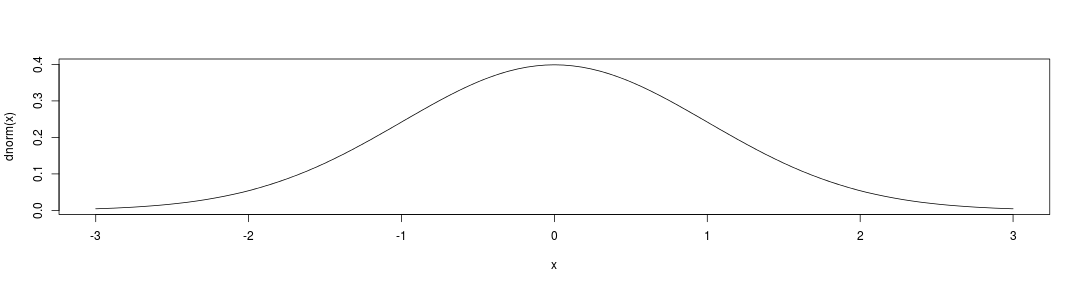### Gaussian cumulative distribution

pnorm(0.5); pnorm(c(-0.5,0,0.5))

 0.6914625

 0.3085375 0.5000000 0.6914625

curve(pnorm, xlim=c(-3,3))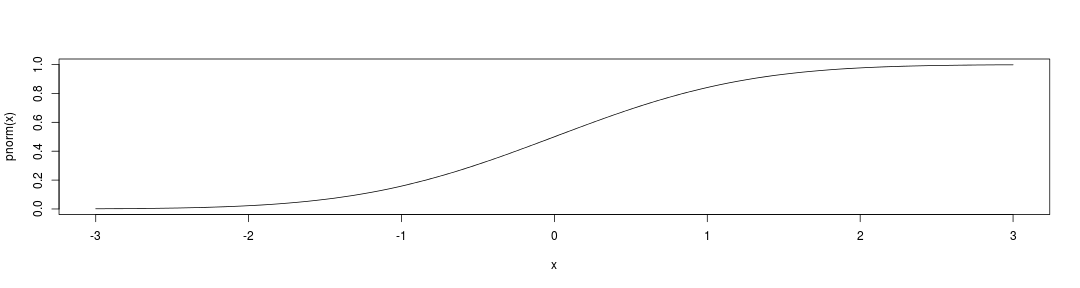### Inverse Gaussian distribution

qnorm(0.975) # quantile of order 97.5%

 1.959964

curve(qnorm, xlim=c(0,1))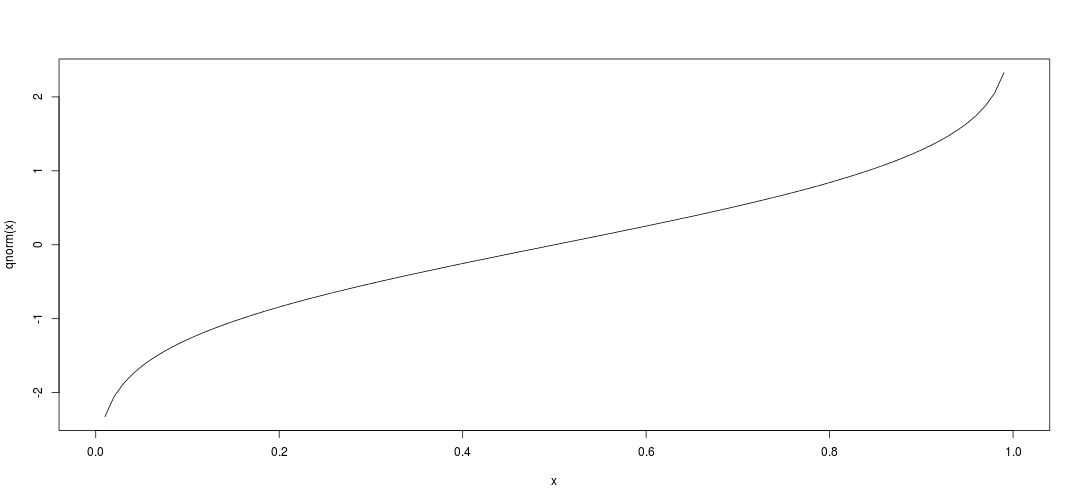### Continuous density distributions

The same functions are available for other continuous distributions, as: beta, Cauchy, exponential, Fisher, gamma, log-normal, uniform,, $$\chi^2$$… see help(Distributions).

### Poisson distribution

dpois(1, lambda=3)

 0.1493612

barplot(dpois(1:10, lambda=3), col="darkred",
names=1:10)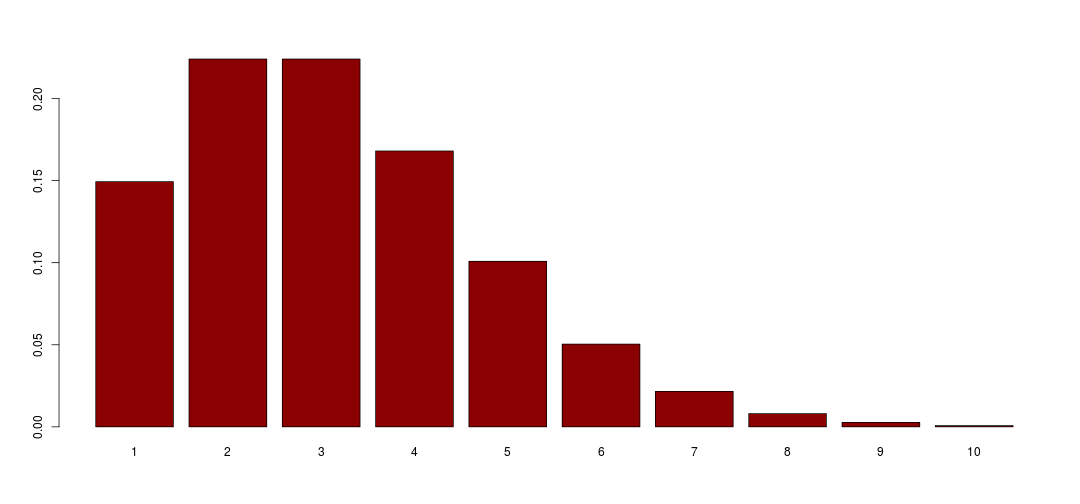### Poisson cumulative distribution

ppois(1, lambda=3)

 0.1991483

barplot(ppois(1:10, lambda=3), col="darkred",
names=1:10)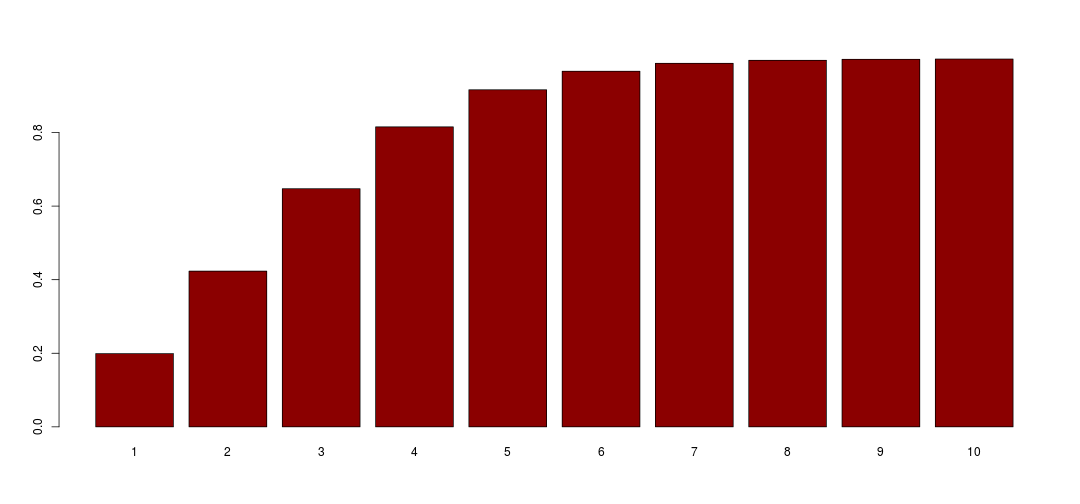### Inverse Poisson distribution

qpois(0.95, lambda=3) # quantile of order 95%

 6

x <- seq(0,1,length=1000)
plot(x, qpois(x,lambda=3), type="h")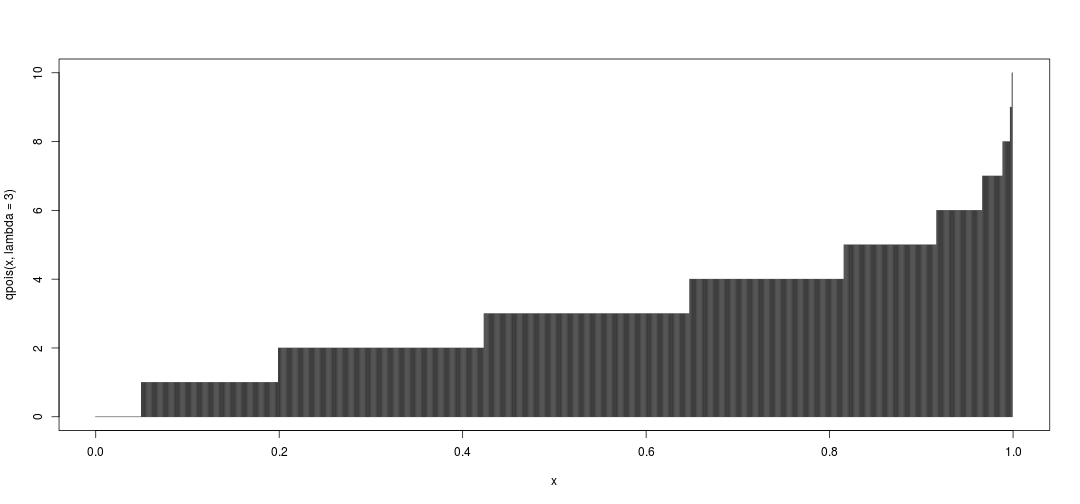### Discrete density distributions

The same functions are available for other discrete distributions, as: binomial, multinomial, negative binomial, … see help(Distributions).

### Random sampling

R can generate pseudo-random numbers or samples. A random sample is taken from a list using the function sample (with or without replacement):

sample(1:12, 5, replace=FALSE)

  1  9  8 12  6

sample(1:12, 20, replace=TRUE)

  12  3  7  2  9  3 11  3  4  7 10  8 12  3  1  8  3  1  7 12


### Random number generation

Random numbers are generated from a given distribution using the prefix “r” with the previously introduced functions:

rnorm(3); hist(rnorm(50, mean=2, sd=3))

  0.1460172 -0.3260648 -1.2325926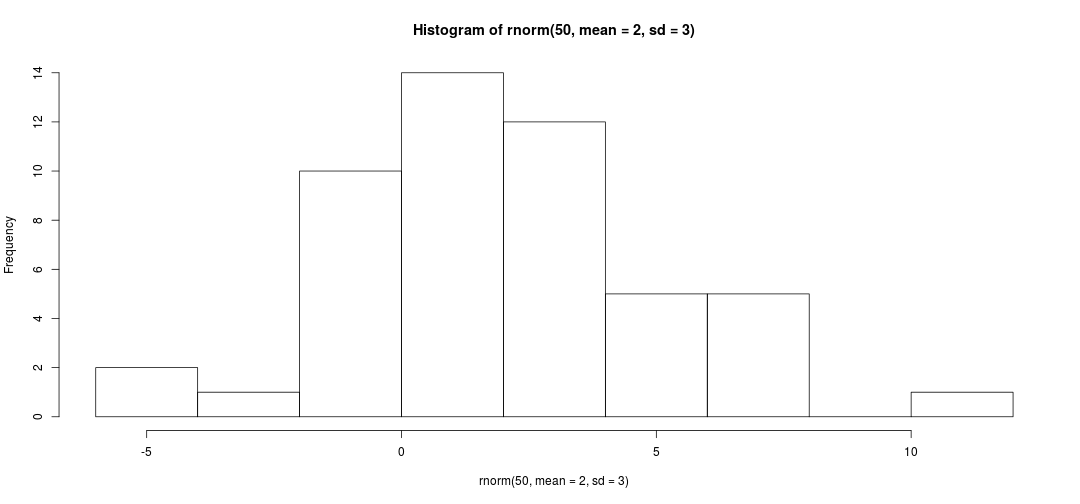### Random number generation

Large samples approximate well the probability distribution:

hist(rnorm(1000), col="darkred", freq=FALSE)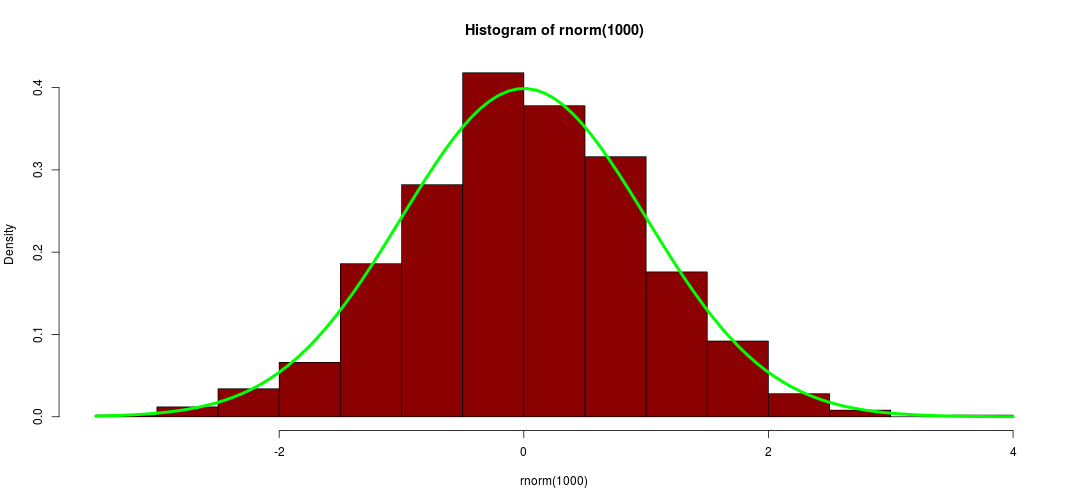### Reproductible random sampling

Random functions produce a different result each time they are called. Reproducible results can be obtained using set.seed with an arbitrary random seed:

rnorm(3) # varies each time it is called

 -0.4628617  1.1976318  2.0578466

set.seed(2807); rnorm(3)

 -1.1624644 -0.1750661  0.8881384


## Statistical tests• normality tests
• equality tests
• independence tests

### Normality tests

Standard tests to check the normality of a sample are the Kolmogorov-Smirnov test and the Shapiro-Wilk test:

to.test <- rnorm(100, mean=5)
shapiro.test(to.test)


Shapiro-Wilk normality test

data:  to.test
W = 0.99396, p-value = 0.9389


### Normality tests

to.test <- runif(100)
res <- ks.test(to.test, "dnorm")
res; res$statistic; res$p.value


One-sample Kolmogorov-Smirnov test

data:  to.test
D = 0.75723, p-value < 2.2e-16
alternative hypothesis: two-sided

        D
0.7572269

 0


### Student test

Student test is used to test an average value:

to.test <- rnorm(100, mean=5)
t.test(to.test, mu=5)


One Sample t-test

data:  to.test
t = 0.49773, df = 99, p-value = 0.6198
alternative hypothesis: true mean is not equal to 5
95 percent confidence interval:
4.847839 5.254059
sample estimates:
mean of x
5.050949


### Student test

Student test is used to test the mean equality between two samples:

to.test <- rnorm(100)
to.test2 <- rnorm(100, mean=1)
res <- t.test(to.test, to.test2, var.equal=TRUE)
res$p.value; res$statistic; qt(c(0.025,0.975), df=99)

 5.562276e-09

        t
-6.096964

 -1.984217  1.984217


### Wilcoxon test

Wilcoxon test is a non parametric test to test the equality between two distributions:

to.test <- rnorm(100); to.test2 <- runif(200)
wilcox.test(to.test, to.test2)


Wilcoxon rank sum test with continuity correction

data:  to.test and to.test2
W = 7163, p-value = 6.208e-05
alternative hypothesis: true location shift is not equal to 0


### Chi square test

$$\chi^2$$ test test the dependancy between two variables in a contingency table:

CT <- as.table(rbind(c(762, 327, 468),
c(484, 239, 477)))
dimnames(CT) <- list(gender = c("M","F"),
party =
c("Democrat","Independent", "Republican"))
res <- chisq.test(CT)  ; res


Pearson's Chi-squared test

data:  CT
X-squared = 30.07, df = 2, p-value = 2.954e-07


### Chi square test

The resulting object is a list with further information:

names(res)

 "statistic" "parameter" "p.value"   "method"    "data.name" "observed"
 "expected"  "residuals" "stdres"

res$expected   party gender Democrat Independent Republican M 703.6714 319.6453 533.6834 F 542.3286 246.3547 411.3166  ## Linear regression• fit a linear regression • interpretation and tests • prediction ### Fit a linear regression data(airquality) res <- lm(Ozone~Wind, data=airquality); res   Call: lm(formula = Ozone ~ Wind, data = airquality) Coefficients: (Intercept) Wind 96.873 -5.551  ### Interpret results names(res)    "coefficients" "residuals" "effects" "rank"  "fitted.values" "assign" "qr" "df.residual"  "na.action" "xlevels" "call" "terms"  "model"  res$coefficients

(Intercept)        Wind
96.872895   -5.550923


### Interpret results

head(res$fitted)   1 2 3 4 6 7 55.79607 52.46551 26.93127 33.03728 14.16414 49.13496  head(res$residuals)

        1         2         3         4         6         7
-14.79607 -16.46551 -14.93127 -15.03728  13.83586 -26.13496

cor(airquality$Ozone, airquality$Wind,
use="complete.obs")

 -0.6015465


### Graphics

R is an object oriented program: the class of res is “lm” that has a dedicated plot function: plot(res) plots four charts

par(mfrow=c(2,2))
plot(res)